# 1.1.16.多項式迴歸：基函式拓展線性迴歸模型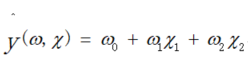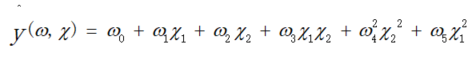（令人驚訝的）觀察到這仍然是一個線性模型：要想到這一點，想象以下建立一個新變數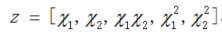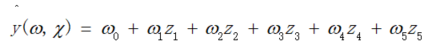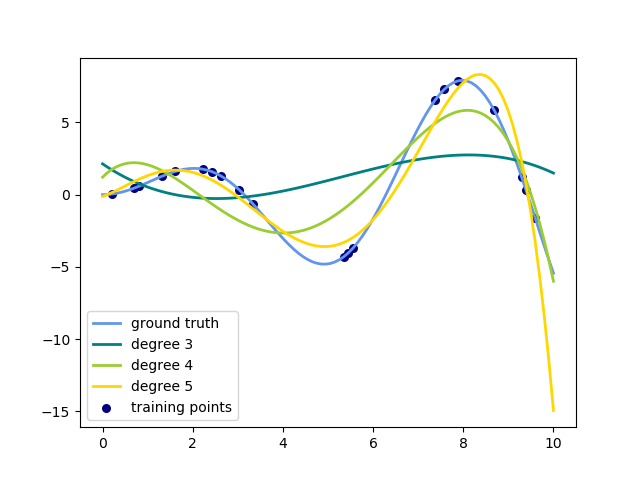```from sklearn.preprocessing import PolynomialFeatures
import numpy as np

X = np.arange(6).reshape(3, 2)
#print(X)#[[0 1] [2 3] [4 5]]

poly = PolynomialFeatures(degree=2)

print(poly.fit_transform(X))#->x1,x2->1,x1,x2,x1^2,x2^2,x1x2
'''[[ 1.  0.  1.  0.  0.  1.]
[ 1.  2.  3.  4.  6.  9.]
[ 1.  4.  5. 16. 20. 25.]]'''```

``````from sklearn.preprocessing import PolynomialFeatures
from sklearn.linear_model import LinearRegression
from sklearn.pipeline import Pipeline
import numpy as np
model = Pipeline([('poly', PolynomialFeatures(degree=3)),('linear', LinearRegression(fit_intercept=False))])
x = np.arange(5)
y = 3 - 2 * x + x ** 2 - x ** 3
model = model.fit(x[:, np.newaxis], y)
#print(model.named_steps['linear'].coef_)[ 3. -2.  1. -1.]
``````

``````from sklearn.linear_model import Perceptron

from sklearn.preprocessing import PolynomialFeatures
import numpy as np
X = np.array([[0, 0], [0, 1], [1, 0], [1, 1]])
y = X[:, 0] ^ X[:, 1]

#print(y)#[0 1 1 0]

X = PolynomialFeatures(interaction_only=True).fit_transform(X).astype(int)
#print(X)
'''[[1 0 0 0]
[1 0 1 0]
[1 1 0 0]
[1 1 1 1]]'''
clf = Perceptron(fit_intercept=False, max_iter=10, tol=None,shuffle=False).fit(X, y)
#print(clf.predict(X))#[0 1 1 0]
#print(clf.score(X,y))1.0
``````